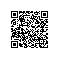# 面试官：JavaScript 原始数据类型 Symbol 有什么用？

const symbol1 = Symbol();
const symbol2 = Symbol();

symbol1 === symbol2; // false

const obj = {};
obj[symbol1] = 'Hello';
obj[symbol2] = 'World';

obj[symbol1]; // 'Hello'
obj[symbol2]; // 'World'

const symbol1 = Symbol();

typeof symbol1; // 'symbol'
symbol1 instanceof Object; // false

// Throws "TypeError: Symbol is not a constructor"
new Symbol();

Symbol()函数只有一个参数，字符串description。这个字符串参数的唯一作用是辅助调试，也就是它的toString()值。但是请注意，两个具有相同description的symbol也是不相等的。

const symbol1 = Symbol('my symbol');
const symbol2 = Symbol('my symbol');

symbol1 === symbol2; // false
console.log(symbol1); // 'Symbol(my symbol)'

const symbol1 = Symbol.for('test');
const symbol2 = Symbol.for('test');

symbol1 === symbol2; // true
console.log(symbol1); // 'Symbol(test)'

JavaScript 内置了一个 symbol ，那就是 ES6 中的Symbol.iterator  。拥有Symbol.iterator函数的对象被称为可迭代对象，就是说你可以在对象上使用for/of 循环。

const fibonacci = {

let a = 1;
let b = 1;
let temp;

yield b;

while (true) {
temp = a;
a = a + b;
b = temp;
yield b;
}

}
};

// Prints every Fibonacci number less than 100
for (const x of fibonacci) {
if (x >= 100) {

break;

}
console.log(x);
}

class MyClass {
constructor(obj) {

Object.assign(this, obj);

}

iterator() {

const keys = Object.keys(this);
let i = 0;
return (function*() {
if (i >= keys.length) {
return;
}
yield keys[i++];
})();

}
}
MyClass 的实例是可迭代对象，可以遍历对象上面的属性。但是上面的类有个潜在的缺陷，假设有个恶意用户给 MyClass 构造函数传了一个带有iterator属性的对象：

const obj = new MyClass({ iterator: 'not a function' });

function getObj() {
const symbol = Symbol('test');
const obj = {};
obj[symbol] = 'test';
return obj;
}

const obj = getObj();

Object.keys(obj); // []

// 除非有这个 symbol 的引用，否则无法访问该属性
obj[Symbol('test')]; // undefined

// 用 getOwnPropertySymbols() 依然可以拿到 symbol 的引用
const [symbol] = Object.getOwnPropertySymbols(obj);
obj[symbol]; // 'test'

const symbol = Symbol('test');
const obj = { [symbol]: 'test', test: symbol };

JSON.stringify(obj); // "{}"使用钉钉扫一扫加入圈子
+ 订阅